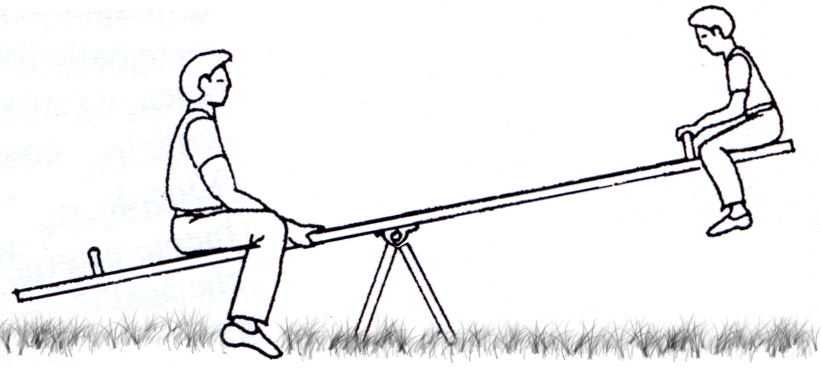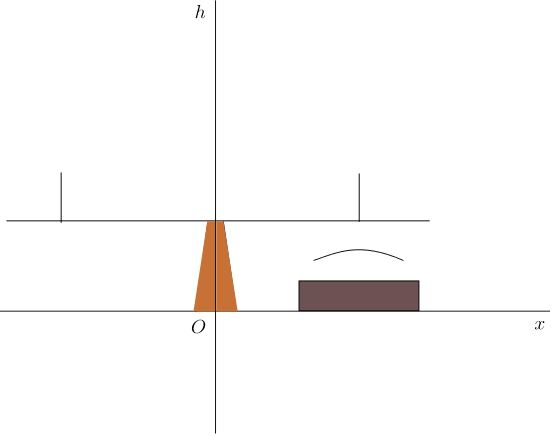# A Trigonometric Model In The Park

Geometry Level 4

Michaelle decided to go to the playground. She saw two boys at the swing set, composed of a board and two seats.Michaelle obtained the following mathematical model for height $h$ above the ground of a seat under which she put a location sensor (in dark brown, picture below):

$h(t)=\displaystyle\dfrac{1}{2}\cdot\left[\sin\left(\dfrac{\pi}{4}t-\dfrac{\pi}{4}\right)\right]+\dfrac{4}{5}$

where $h$ is expressed in metres and time $t$ in seconds.If the period of this motion is $A$, the initial distance from the ground to the seat is $B$, and the center of oscillation is $C$, what is $A+B+C$ (rounded to the nearest hundredth)?

×